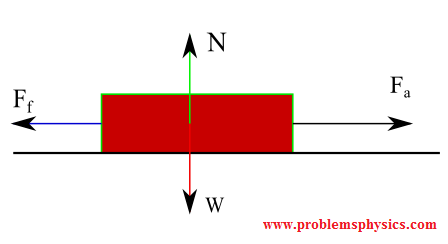# An object with a mass of 6 kg is on a surface with a kinetic friction coefficient of  8 . How much force is necessary to accelerate the object horizontally at  7 m/s^2?

Feb 18, 2018

Consider,where ${\mu}_{k} = 8.0$ (which is like super glue!).

Recall Newton's second law,

$\Sigma {F}_{y} = {F}_{N} - m g = 0$
$\implies {F}_{N} = m g$

$\therefore {F}_{N} = 6 k g \cdot \frac{9.8 m}{s} ^ 2 = 58.8 N$

Hence,

$\Sigma {F}_{x} = {F}_{a} - {F}_{\text{fr}} = m a$

$\implies {F}_{a} - {\mu}_{k} \cdot {F}_{N} = m a$

$\implies {F}_{a} = m \left(a + {\mu}_{k} \cdot {F}_{N}\right)$

$\therefore {F}_{a} = 6 k g \cdot \left(\frac{7 m}{s} ^ 2 + 8.0 \cdot 58.8 N\right) \approx 3 k N$

That's some serious work we need to do!